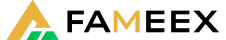Register
Support Center

Visit our Support Center. It's the easiest way to access all the crypto information needed.

FAMEEX provides a user-friendly, secure, reliable, and excellent crypto trading experience. Download our mobile APP and Trade your Bitcoin anywhere and whenever you want!Go trade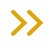Support CenterContractsHow to Calculate your PnL
How to Calculate your PnL
2022/06/01 12:29:45

Our tutorial video provides quick access to solutions for common problems and information regarding related topics:

More details are available in the whole article below.

For every user who is involved in U-Futures Trading, there is an independent futures wallet, which will be used for margin and balance calculation.

1. Terms and Explanations of Futures Wallet2. Terms and Explanations  of Positions3. How to Calculate your PnL?

Realized Profit and Loss is the revenue or loss for users after the positions are closed.

(1) Realized PnL (after position closed)

If you open only one direction of long/short positions:

Realized PnL = sum{The Amount of Close Position X (Close Price - Avg. Open Price)}

For example, a user opens a long position in the amount of 2 BTC at the price of 500 USDT, and then closes it in the amount of 1 BTC at the price of 1,000 USDT.

The Realized PnL = 1 X (1,000-500) = 500 USDT

If you close one direction of long/short positions:

Realized PnL = sum{The Amount of Close Position X (Avg. Open Price - Close Price)}

For example, a user closes a short position in the amount of 10 BTC at the price of 500 USDT and then opens in the amount of 8 BTC at the price of 1,000 USDT.

The Realized PnL = 8 X (500-1,000) = -4,000 USDT

(2) Unrealized PnL (Positions size PnL)

Long Position of Unrealized PnL = (The Last Mark Price - Avg. Open Price) X Position Size

Short Position of Unrealized PnL = (Avg. Open Price - The Last Mark Price) X Position Size

4. Average Price

Average Price = (Position Amount X Avg. Price + New Position Amount X Avg. Open Price) / (Petition Amount + New Position Amount)

New Avg. Open Price = (Filled Position 1 X Filled Price 1 + Filled Position 2 X Filled Price 2 + …) / New Position Amount

New Position Amount = Open Position Amount of Filled Price 1 + Open Position Amount of Filled Price 2 + ...

For example, the last mark price is 600 USDT. A user holds 6 BTC with a long position at the avg. open price of 500 USDT, then buys 5 BTC of the long position.

The filled price for the 5 BTC is as follows:The 5 BTC Avg. Filled Price = (1 X 580 + 1 X 570 + 3 X 560) / 5 = 566 USDT

New Avg. Position Price = (6 X 500 + 5 X 566) / (6 + 5) = 530 USDT

New Position Amount = 5 + 6 = 11 BTC

5. Estimated Liquidation Price

(1) Estimated Liquidation Price for Cross Margin

If the Estimated Liquidation Price (ELP) for Cross Margin is X, its formula is:

[Account Balance - Isolated Margin + (ELP - Average Position Price) X Size of Long Position + (Average Position Price - ELP) X Size of Short Position + Unrealized PnL - Maintenance Margin of Cross Contract ] / (Size of Long Position X ELP + Size of Short Position X ELP ) = Maintenance Margin Rate

(2) Estimated Liquidation Price of Isolated Margin

If the Estimated Liquidation Price (ELP) for Isolated Margin is X, its formula is:

[ Isolated Margin + (ELP - Average Position Price) X Size of Long Position + (Average Position Price - ELP) X Size of Short Position] /  (Size of Long Position X ELP + Size of Short Position X ELP) = Maintenance Margin Rate

6. Bankruptcy Price

Traders need to keep a certain percentage of their fund as the maintenance margin. If their position margin is less than the maintenance margin, the auto deleveraging system or liquidation will be triggered. Our system will close their positions at bankruptcy price but the actual filled price depends on the actual orders.

(1) Bankruptcy Price in Cross Mode (BPC)

Bankruptcy Price in Cross Mode = Account Balance - Isolated Margin + Unrealized PnL - Maintenance Margin of Cross Contract +(BPC - Avg. Open Price) X Size of Long Position + (Avg. Open Price - BPC) X Size of Short Position - Size of Long Position X BPC X Transaction Fee Rate of Taker - Short of Position X BPC X Transaction Fee Rate of Taker = 0

(2) Bankruptcy Price in Isolated Mode (BPI)

Bankruptcy Price in Isolated Mode = Isolated Margin + (BPI - Avg. Open Price) X Size of Long Position + (Avg. Open Price - BPI) X Size of Short Position - Size of Long Position X BPC X Transaction Fee Rate of Taker - Short of Position X BPI X Transaction Fee Rate of Taker = 0

7. Return on Equity Rate

ROE% of Cross Margin = Unrealized PnL / Cost of Margin X 100%

ROE% of Isolated Margin = Unrealized PnL / (Mark Price X Position / Leverage) X 100%

•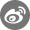•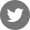•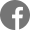•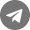•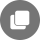Related Articles
Bugs Report:Safe@mail.fameex.info